22.12.2017

# Creating animated SVG without third party tools (easily made morph animations only with frame cloning)

Actually, at the start, you need some SVG editor. But no animation support needed. Recommend perfect Inkscape, which is suitable vector editor and as the benefit, it works directly with SVG files.

1. create/copy your drawing which will be animated (the whole drawing must be in one layer)
2. convert all non-path elements to paths
3. duplicate main layer for each step of animation (but only significant steps - exact motion between frame A and frame B will be interpolated)
4. modify paths in each animation frames

Restrictions on frame modification:

1. cannot change element drawing priority
2. cannot add or remove elements
3. cannot add or remove new points in paths

So basically is only about path changing. You can move paths freely, rotate them, scale them, or change points parameters. Any essential changes in main drawing discards prepared animation and it must be created again. So when starting with animating the drawing should be finished.

Example

Create some drawing: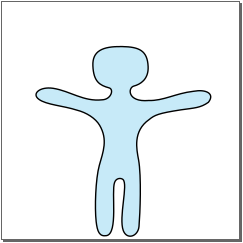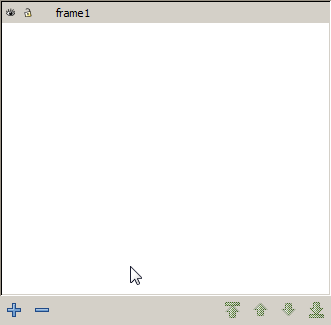Make first animation step (duplicate the first frame and modify):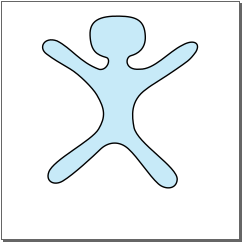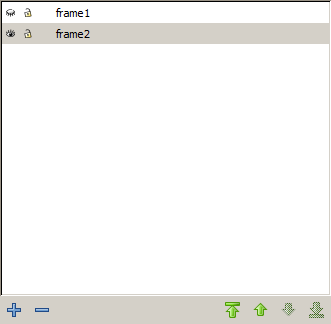Make second step (it's ok to duplicate frame2):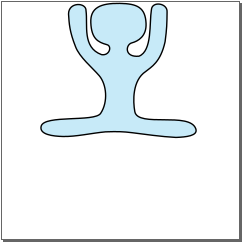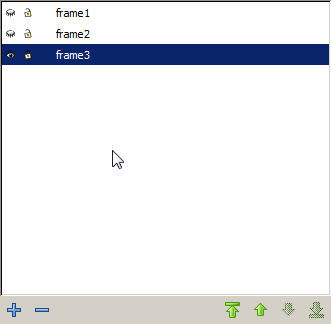After some minor changes in each frame here is generated animation:I recommend to continuously debug animation and update paths in all frames which needed. But keep in mind mentioned restrictions - you can break the animation easily.

Animation generation logic

The SVG is XML file and you can just simply grab all paths in layers and put them as an animation sequence. Here is code in PHP - but it's not complex so you can rewrite it in the desired language.

``````header("Content-type: image/svg+xml");

// file query
\$q = \$_GET['q'];

// animation flag (default true)
\$is_anim = isset(\$_GET['anim']) ? \$_GET['anim'] == 1 : true;

// flag to repeat only once
\$is_once = isset(\$_GET['once']) && \$_GET['once'] == 1;

// flag to return back in sequence to main frame (default true)
\$is_back = isset(\$_GET['back']) ? \$_GET['back'] == 1 : true;

// frame duration in seconds (default 1 sec)
\$dur = isset(\$_GET['dur']) ? doubleval(\$_GET['dur']) : 1;

// index of main layer (default 0 - first)
\$main_layer_idx = isset(\$_GET['main']) ? intval(\$_GET['main']) : 0;

if ((\$xml = @new SimpleXMLElement(file_get_contents(\$q))) != null) {

\$xml["preserveAspectRatio"] = "none";

\$layers = array();

// iterate trought layers
foreach (\$xml->g as \$node) {
\$attrs = \$node->attributes('inkscape', true);

if (strval(\$attrs['groupmode']) == 'layer') {

// collect all layers sorted by name
\$layer = array(
'node' => \$node,
'name' => strval(\$attrs['label'])
);

\$brk = false;
\$pos = 0;

while (!\$brk && \$pos < count(\$layers)) {
if (strcasecmp(\$layer['name'], \$layers[\$pos]['name']) < 0) {
\$layers[] = null;

for (\$sf = count(\$layers) - 1; \$sf > \$pos; \$sf--) {
\$layers[\$sf] = \$layers[\$sf - 1];
}

\$layers[\$pos] = \$layer;

break;

} else {
\$pos++;
}
}

if (\$pos == count(\$layers)) {
\$layers[] = \$layer;
}
}
}

// set main layer to be visible
\$layers[\$main_layer_idx]["node"]["style"] = "display:inline";

// force other layers to be hidden
for (\$sf = 0; \$sf < count(\$layers); \$sf++) {
if (\$sf != \$main_layer_idx) {
\$layers[\$sf]["node"]["style"] = "display:none";
}
}

\$paths = array();

// collect paths in main layer
foreach (\$layers[\$main_layer_idx]["node"]->children() as \$node) {
if (\$node->getName() == 'path') {
\$attrs = \$node->attributes();

\$paths[] = array(
"node" => \$node,
"ds" => array(strval(\$attrs["d"])) // the path data
);
}
}

for (\$sf = 0; \$sf < count(\$layers); \$sf++) {
if (\$sf != \$main_layer_idx) {
\$idx = 0;

foreach (\$layers[\$sf]["node"]->children() as \$node) {
if (\$node->getName() == 'path') {
\$attrs = \$node->attributes();

\$paths[\$idx]["ds"][] = strval(\$attrs["d"]); // stack path state in another frame

\$idx++;
}
}
}
}

if (\$is_anim) {

// process the animation
for (\$sf = 0; \$sf < count(\$paths); \$sf++) {

\$ds_put = '';
\$idx = 0;

// concat path states
while (\$idx < count(\$paths[\$sf]["ds"])) {
\$ds_put .= (\$ds_put == false ? '' : ';') . \$paths[\$sf]["ds"][\$idx];
\$idx++;
}

if (\$is_back) {
\$idx -= 2;

// concat path states to back
while (\$idx >= 0) {
\$ds_put .= (\$ds_put == false ? '' : ';') . \$paths[\$sf]["ds"][\$idx];
\$idx--;
}
}

// build animation tag
\$tag_value = 'animate dur="' . \$dur . 's" ';
\$tag_value .= 'repeatCount="' . (\$is_once ? '1' : 'indefinite') . '" ';
\$tag_value .= 'attributeName="d" ';
\$tag_value .= 'fill="freeze" ';
\$tag_value .= 'calcMode="linear" ';
\$tag_value .= 'values="' . \$ds_put . '"';

// and append to path node in main layer
}
}

for (\$sf = 0; \$sf < count(\$layers); \$sf++) {
if (\$sf != \$main_layer_idx) {
unset(\$layers[\$sf]['node']);
}
}

// output the xml
echo \$xml->asXML();

}
``````

Other examples

Some concepts could be made really easily. For example, rotating gears - just duplicate frame and rotate a bit around wheel axis:Morphing things - be sure you have enough path points to create each required shape:Or animation made by simply rotating and scaling:© 2021 Dzejkob games | info@dzejkobgames.eu